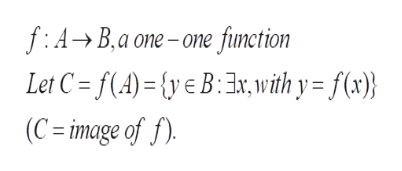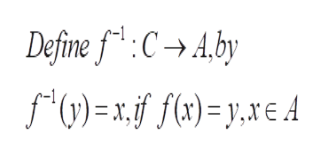Suppose f is a one-to-one function with domain A andrange B. How is the inverse functiondefined? What is the domain and range of the inverse function

Question

Suppose f is a one-to-one function with domain A and
range B. How is the inverse function
defined? What is the domain and range of the inverse function

Step 1

To define the inverse of a function (assuming its existence)

Step 2

The inverse of f can only be defined if f is one-one .help_outlineImage Transcriptionclosef:A- B.a one-one fiunction Let C = f(A)= {y€B3x, with y= f(x)} (C image of f) fullscreen
Step 3

Note that the inverse of f is defined (in general ...help_outlineImage TranscriptioncloseDefine f CAby *(y) = x. 1f f(x)= y,xE A fullscreen

Want to see the full answer?

See Solution

Want to see this answer and more?

Our solutions are written by experts, many with advanced degrees, and available 24/7

See Solution
Tagged in

Calculus